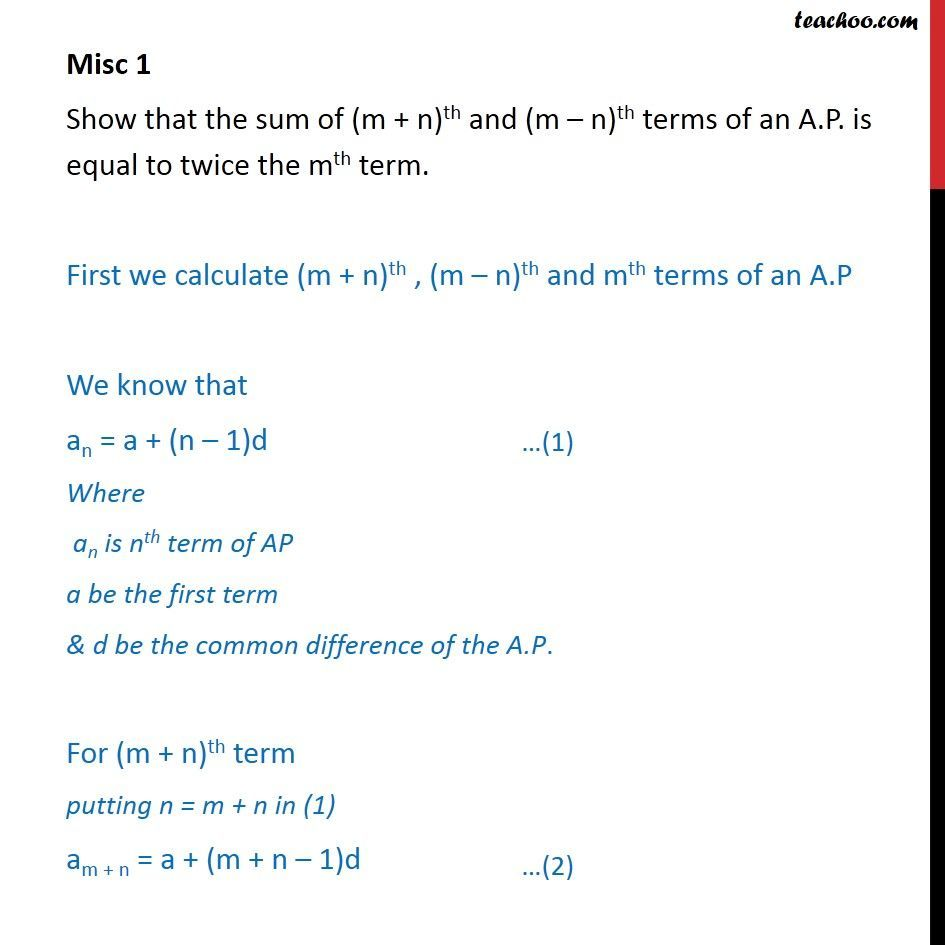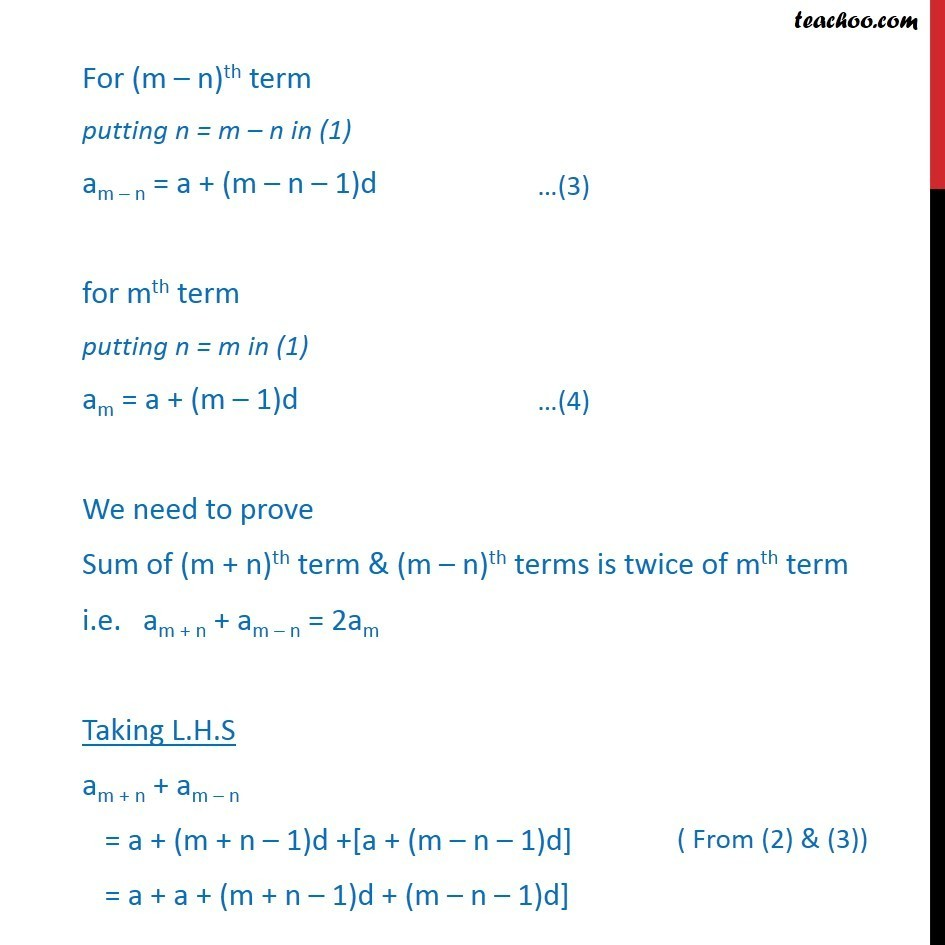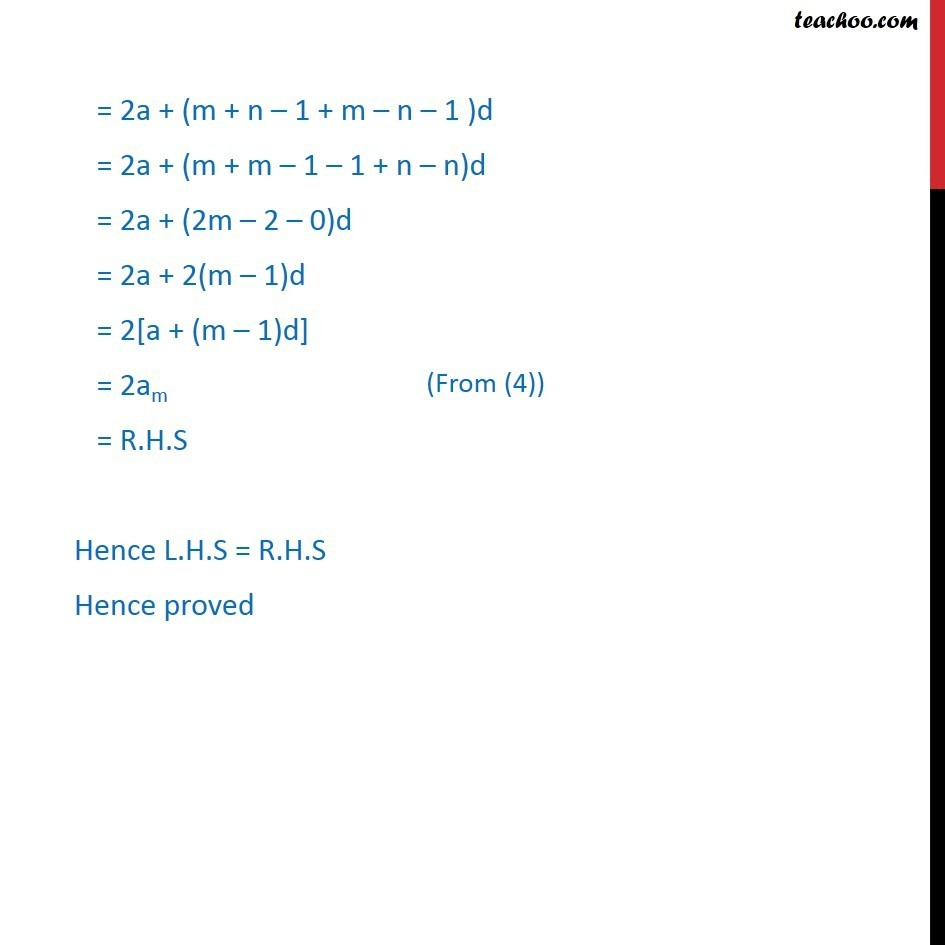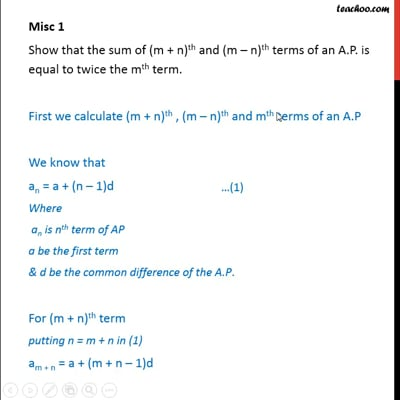Miscellaneous

Chapter 9 Class 11 Sequences and Series
Serial order wiseThis video is only available for Teachoo black users

Solve all your doubts with Teachoo Black (new monthly pack available now!)

### Transcript

Misc 1 Show that the sum of (m + n)th and (m – n)th terms of an A.P. is equal to twice the mth term. First we calculate (m + n)th , (m – n)th and mth terms of an A.P We know that an = a + (n – 1)d Where an is nth term of AP a be the first term & d be the common difference of the A.P. For (m + n)th term putting n = m + n in (1) am + n = a + (m + n – 1)d For (m – n)th term putting n = m – n in (1) am – n = a + (m – n – 1)d for mth term putting n = m in (1) am = a + (m – 1)d We need to prove Sum of (m + n)th term & (m – n)th terms is twice of mth term i.e. am + n + am – n = 2am Taking L.H.S am + n + am – n = a + (m + n – 1)d +[a + (m – n – 1)d] = a + a + (m + n – 1)d + (m – n – 1)d] = 2a + (m + n – 1 + m – n – 1 )d = 2a + (m + m – 1 – 1 + n – n)d = 2a + (2m – 2 – 0)d = 2a + 2(m – 1)d = 2[a + (m – 1)d] = 2am = R.H.S Hence L.H.S = R.H.S Hence proved